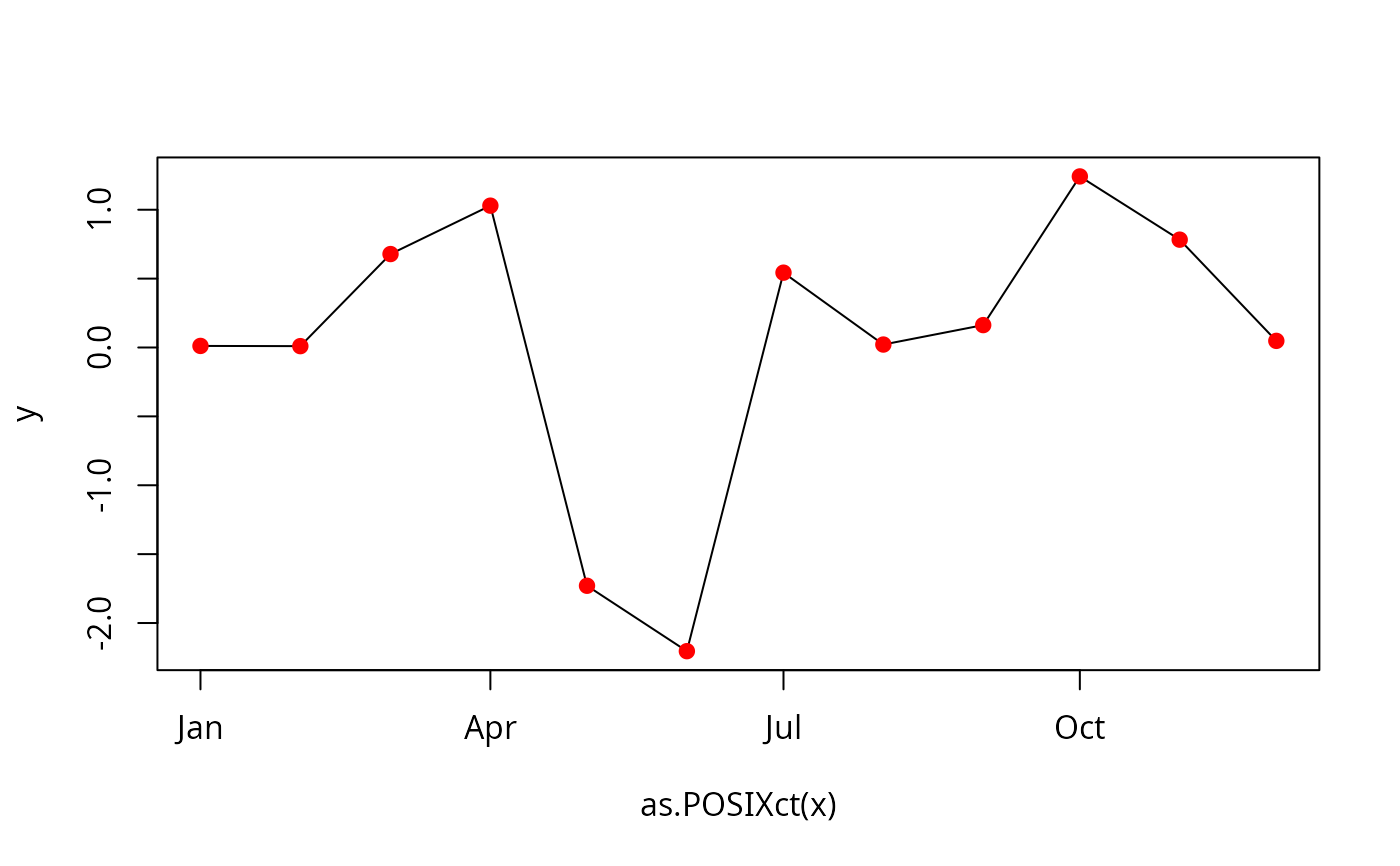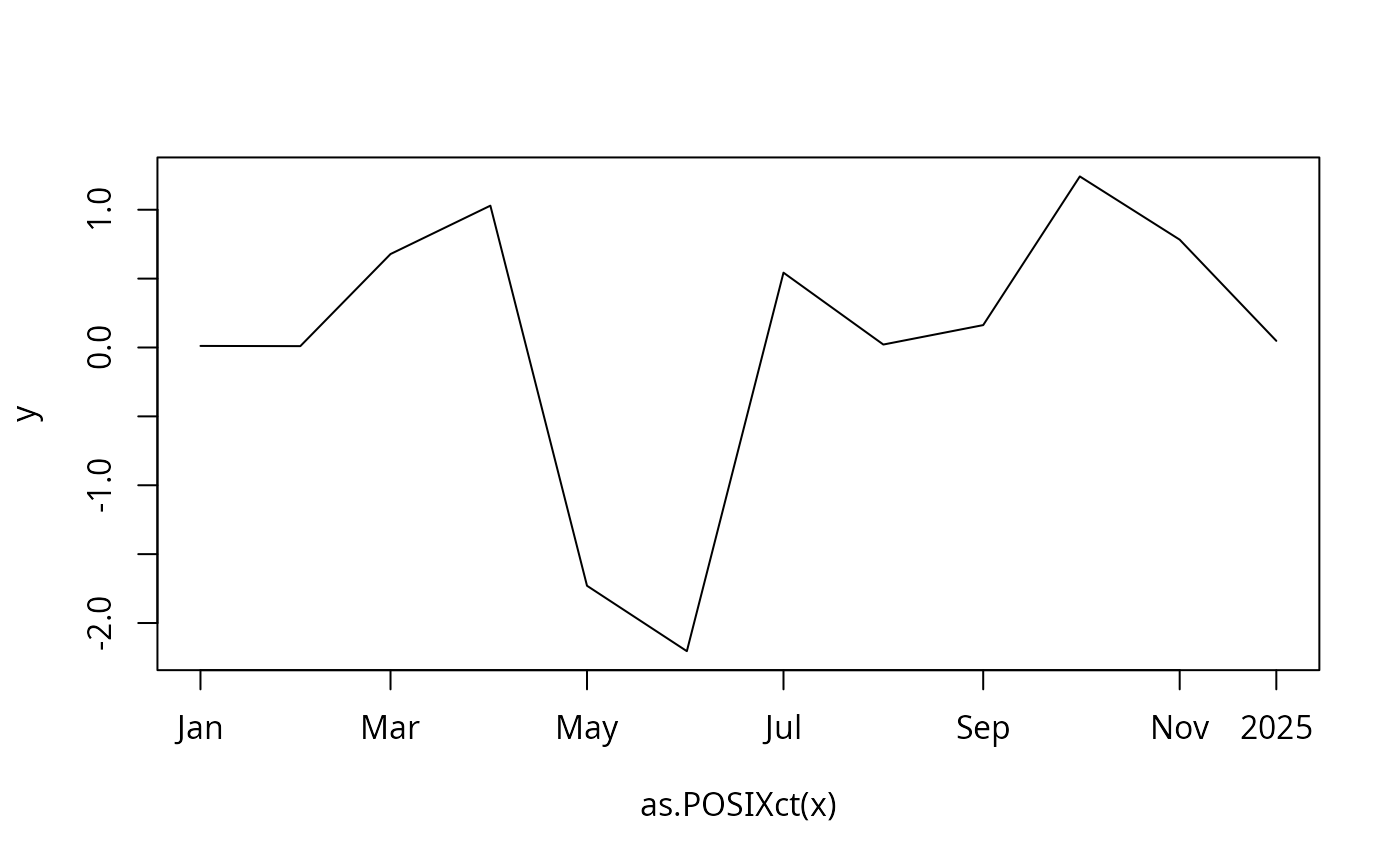Plot methods for "timeDate" objects.

## Usage

# S4 method for timeDate
plot(x, y, ...)
# S4 method for timeDate
lines(x, y, ...)
# S4 method for timeDate
points(x, y, ...)

axis.timeDate(side, x, at, format = NULL, labels = TRUE, ...)

# S3 method for timeDate
pretty(x, n=5, min.n=n%/%3, shrink.sml=0.75,
high.u.bias=1.5, u5.bias=0.5+1.5*high.u.bias,
eps.correct=0, ...)

## Arguments

x, y, at

an object of class timeDate.

side

an integer specifying which side of the plot the axis is to be drawn on. The axis is placed as follows: 1=below, 2=left, 3=above and 4=right.

format

a POSIX format string, e.g. "%Y-%m-%d".

labels

either a logical value specifying whether annotations are to be made at the tickmarks, or a vector of character strings to be placed at the tickpoints.

n

an integer giving the desired number of intervals.

min.n

a nonnegative integer giving the minimal number of intervals.

shrink.sml

a positive numeric by a which a default scale is shrunk in the case when range(x) is very small.

high.u.bias

a non-negative numeric, typically > 1. Larger high.u.bias values favor larger units.

u5.bias

a non-negative numeric multiplier favoring factor 5 over 2.

eps.correct

an integer code, one of 0,1,2. If non-0, a correction is made at the boundaries.

...

arguments passed to other methods.

## Value

returns a summary report of the details of a "timeDate"

object. This includes the starting and end date, the number of dates the format and the financial center in use.

## Examples

## timeCalendar -
x <- timeCalendar()
y <- rnorm(12)

## Plotting :

plot(x, y, type = "l")
points(x, y, pch = 19, col = "red")plot(x, y, type = "l", xaxt = "n")
axis.timeDate(1, at = x[c(1, 3, 5, 7, 9, 11)], format = "%b")
axis.timeDate(1, at = x, format = "%Y")